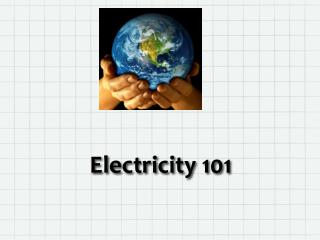DownloadDownload PresentationElectricity 101

# Electricity 101

Télécharger la présentation## Electricity 101

- - - - - - - - - - - - - - - - - - - - - - - - - - - E N D - - - - - - - - - - - - - - - - - - - - - - - - - - -
##### Presentation Transcript

1. Electricity 101

2. What is Electricity? • Electricity is a type of kinetic energy characterized by the flow of charged particles. Energy is the ability to do work (or damage), so essentially, it is the movement, or push, of these particles which carries out the work. • Normally, these particles are electrons, the negatively charged subatomic particles found in all atoms. • Important terms to understand are: • Voltage • Current • Resistance • Wattage

3. Voltage • Voltage is the term used for electrical potential, as in potential energy. It is a feature of an electrical field which describes the potential difference between two points. The greater the potential difference, the greater will be the tendency for electrons to flow. It is sometimes described as electrical tension or electrical push. It is measured in VOLTS. • Common voltages for our understanding are: • 110 volts is the common US household electricity • 220 volts is the voltage of certain appliances and also the common voltage inn some other countries • 12 volts is the voltage of auto batteries • 1.5 volts is the voltage of most common portable batteries

4. Current • Current works in electricity just like it works in rivers or in pipes carrying water; it is essentially the rate of flow of the electrons through the conductor. • The more electrons which flow past a certain point in a given period of time, the more electrical energy or work which can be done. • Current is measured in AMPERES (or AMPS, for short) • Current can be AC (alternating) or DC (direct). Household electricity is AC, battery powered electricity is DC. • We all use “black boxes” which convert AC to DC and some of us use devices called inverters to change DC to AC

5. Resistance • Resistance is like electrical friction. As Electrons flow through a conductor (i.e. a wire), there is friction which impedes their movement. • Just as with other friction, some of the electrical energy is converted to other forms of energy (heat and light) along the way. • Pumping electrons through certain high-resistance materials (such as tungsten wires) can generate heat (i.e. cooking elements) and light (i.e. incandescent light bulbs) • In wiring, the general pattern is that the thicker the wire, the less resistance there will be. Just envision the friction of water flowing through a 6 inch pipe as compared to a 1 inch pipe.

6. Wattage • Wattage is the measurement of electrical POWER. We think of it most often in terms of the brightness of light bulbs, but all electrical devices have a wattage rating printed on their specifications plate. • We measure our electricity use in instantaneous watts or kilowatts (kW) and we are billed in kW-h or kilowatt hours by the utility company. • We discuss power plant capacities in megawatts (MW) or gigawatts (GW), the maximum instantaneous output of the plant.

7. Important Relationships • Voltage = Resistance x Current • Wattage = Voltage x Current • Wattage = Current2x Resistance • Wattage = Voltage2 / Resistance

8. Electric Motors and Generators • MOTOR - When we run electric current through coils of wires in a circular magnetic field, we can cause the wire coils to spin a shaft on which the coils are wrapped. When we connect that spinning shaft to gears, belts, etc. we can use the work of the motor to do a job for us. • GENERATOR – This is simply the same process in reverse; spinning the shaft with some external force (i.e. a steam turbine, water, wind) generates electric current.

9. Batteries A battery is a chemical system of two cells, one of which uses an oxidation reaction to give off electrons and the other of which uses a reduction reaction to absorb the electrons. When we connect the two cells of the battery with a conducting circuit, electric current flows. When the reactants of either of the chemical systems run out, the reactions stop and the battery is “dead”. Rechargeable batteries (car, cell phone, computer) use reversible reaction which, when a current is sent backwards through them, reconstitute the reactants so that the battery is recharged and once again useful. Batteries are best though of as a storage of energy.

10. Electrical Circuits • Series • Additive voltage and constant current • Parallel • Constant voltage and variable current